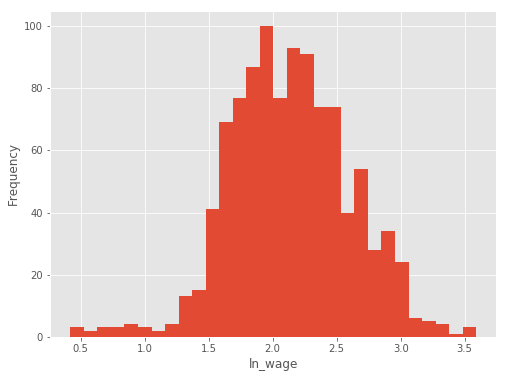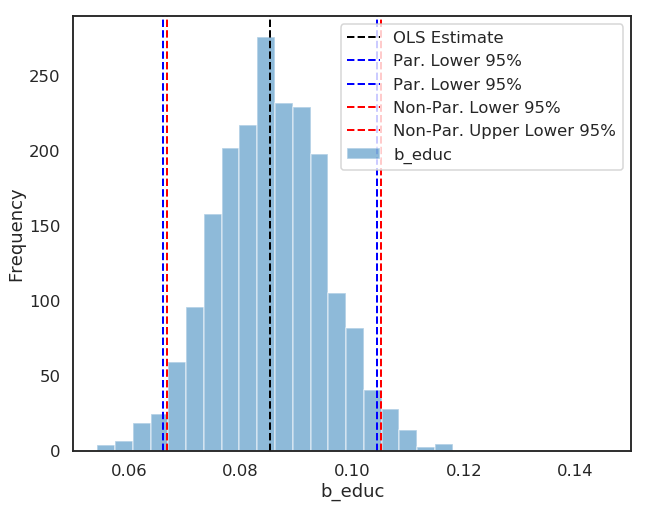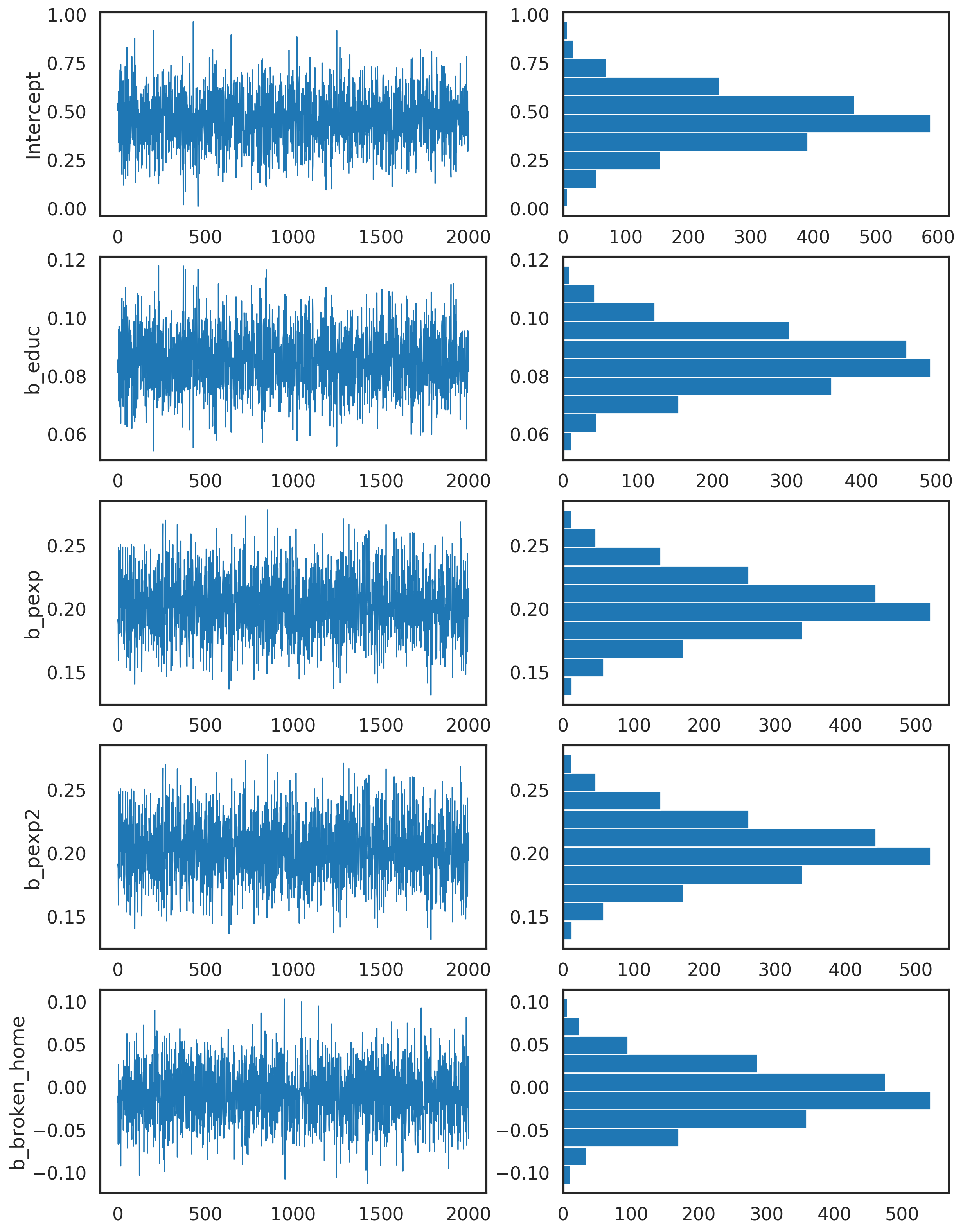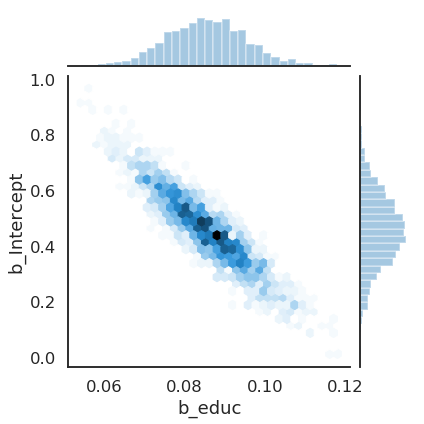# Bayesian Econometrics Introduction

## Introduction to Bayesian Econometrics¶

This course will cover the application of Bayesian statistical methods for econometric inference. Broadly speaking we will

1. Briefly discuss sampling methods for classical statistics
2. Introduce Bayes Rule and Provide an Application
3. Examine the use of Monte Carlo Markov Chains
• Metropolis-Hastings and other Samplers
• Chain "convergence" and diagnostics
4. Application: OLS, Time-Series Econometrics, Heirarchical Models

The frequentist paradigm, arguably the dominant statistical paradigm in the social sciences (and what you all have studied), relies on the following notions:

• $\beta$ is not random but neither is it known. It is a fixed quantity.
• To uncover information about $\beta$, we observe part of some process (e.g. $\mathbf{y=x\beta+\epsilon}$).
• For statistical inference, we rely on repeated trials of $\mathbf{y}$ and $\mathbf{x}$, even if this repetition rarely (if ever) occurs in the social science context.
• $\mathbf{y}$ and $\mathbf{x}$ are considered random
• The model typically attempts to uncover information about $\mathbf{\beta}$ by examining the likelihood function $$prob(\mathbf{y}|\mathbf{b},\mathbf{x})$$ where $\mathbf{b}$ are our estimates of $\beta$

The bayesian paradigm tackles the issue of estimating $\beta$ by

• Treating $\beta$ as random and unknown
• Treating $\mathbf{y}$ and $\mathbf{x}$ as fixed and non-random (at least once they are recorded in your dataset)
• Uncovers information about $\mathbf{\beta}$ by examining the posterior likelihood $$prob(\mathbf{b}|\mathbf{y},\mathbf{x})$$ where $\mathbf{b}$ are our estimates of $\beta$

In quite a lot of instances, these two approaches give you the same estimate for $\beta$. Until recently, Bayesian Statistical modeling wasn't used because calculating the posterior likelihood was computationally challenging, but recent advances in the theory and construction of Monte Carlo Markov Chains and computational ability has really opened the door for Bayesian analysis for problems that might not be estimated using the frequentist paradigm (ie. Maximim Likelihood). There is an ongoing holy war in the two statistical camps, during the semester I will attempt to highlite the pros and cons of each paradigm without taking a position on which one is better. My philosophy is that if it gets the job done, use it while being aware of limitations and advantages.

### Repeated trials in frequentist statistics¶

A good jumping off point for this course is to understand the use of sampling techniques in a classical statistical paradigm.

• Underlying all statistical inference that you have learned in statistics and econometrics is the idea of repeated trials. Bootstrapping highlites this really well.
• We will begin with an exploration of bootstrapping and see the implementation steps.
In :
#load python libraries for this ipython notebook:
%matplotlib inline
import numpy as np
import pandas as pd
import matplotlib.pyplot as plt
import seaborn as sbn
import statsmodels.formula.api as smf
import warnings
warnings.filterwarnings('ignore')

plt.style.use('ggplot')

np.random.seed(12578)

##### Load Tobias and Koop data for time==4¶
In :
tobias_koop=pd.read_csv('https://rlhick.people.wm.edu/econ407/data/tobias_koop_t_4.csv')

In :
tobias_koop.head()

Out:
id educ ln_wage pexp time ability meduc feduc broken_home siblings pexp2
0 4 12 2.14 2 4 0.26 12 10 1 4 4
1 6 15 1.91 4 4 0.44 12 16 0 2 16
2 8 13 2.32 8 4 0.51 12 15 1 2 64
3 11 14 1.64 1 4 1.82 16 17 1 2 1
4 12 13 2.16 6 4 -1.30 13 12 0 5 36
In :
tobias_koop.describe()

Out:
id educ ln_wage pexp time ability meduc feduc broken_home siblings pexp2
count 1034.000000 1034.000000 1034.000000 1034.000000 1034.0 1034.000000 1034.000000 1034.000000 1034.000000 1034.000000 1034.000000
mean 1090.951644 12.274662 2.138259 4.815280 4.0 0.016596 11.403288 11.585106 0.169246 3.200193 27.979691
std 634.891728 1.566838 0.466280 2.190298 0.0 0.920963 3.027277 3.735833 0.375150 2.126575 22.598790
min 4.000000 9.000000 0.420000 0.000000 4.0 -3.140000 0.000000 0.000000 0.000000 0.000000 0.000000
25% 537.250000 12.000000 1.820000 3.000000 4.0 -0.550000 11.000000 10.000000 0.000000 2.000000 9.000000
50% 1081.500000 12.000000 2.120000 5.000000 4.0 0.170000 12.000000 12.000000 0.000000 3.000000 25.000000
75% 1666.500000 13.000000 2.450000 6.000000 4.0 0.720000 12.000000 14.000000 0.000000 4.000000 36.000000
max 2177.000000 19.000000 3.590000 12.000000 4.0 1.890000 20.000000 20.000000 1.000000 15.000000 144.000000
In :
# explore distribution of ln_wage (our dependent variable)
plt.figure(figsize=(8,6))
tobias_koop.ln_wage.plot(kind='hist',bins=30)
plt.xlabel('ln_wage')
plt.show()In :
# run OLS regression:
formula = 'ln_wage ~educ + pexp + pexp2 + broken_home'

mod = smf.ols(formula,data=tobias_koop)
res=mod.fit()
res.summary()

Out:
Dep. Variable: R-squared: ln_wage 0.166 OLS 0.163 Least Squares 51.36 Mon, 28 Jan 2019 1.83e-39 09:02:30 -583.66 1034 1177. 1029 1202. 4 nonrobust
coef std err t P>|t| [0.025 0.975] 0.4603 0.137 3.353 0.001 0.191 0.730 0.0853 0.009 9.179 0.000 0.067 0.104 0.2035 0.024 8.629 0.000 0.157 0.250 -0.0124 0.002 -5.438 0.000 -0.017 -0.008 -0.0087 0.036 -0.244 0.807 -0.079 0.061
 Omnibus: Durbin-Watson: 55.892 1.761 0 112.05 -0.355 4.66e-25 4.448 391

Warnings:
 Standard Errors assume that the covariance matrix of the errors is correctly specified.

These standard errors aren't robust (although we could ask for that). As we saw in Cross-Section, we can non-parametrically bootstrap confidence intervals of our parameters. While bootstrapping is implemented in many ols routines, we implement them manually to see how it works:

Steps for bootstrapping:

1. Loop $R$ times ($R$ = # of boostrapped replicates)
2. For each $r\in R$, sample $N$ times with replacement from rows in $\mathbf{Y}$ and $\mathbf{X}$, noting that $N$ is equal to the number of observations in the original dataset $\mathbf{Y}$ and $\mathbf{X}$. Denote each of these samples as $\mathbf{X}_r$ and $\mathbf{Y}_r$.
3. Estimate an OLS model and recover estimate for replicate $r$: $\hat{\beta}_r = (\mathbf{X}_r'\mathbf{X}_r)^{-1}\mathbf{X}_r'\mathbf{Y}_r$
4. Store results
5. Calculate the standard deviation (our estimate for the standard errors) of each parameter estimate, or construct non-parametric confidence intervals (preferred)

Note, for our purposes we don't need to see the R regression results. We only need to store them and move on to the next replicate. Let's investigate further the res object we created above.

##### How to Recover OLS Estimates from Statsmodels¶
In :
# The parameter values can be accessed here
beta = res.params
print(beta)
print("\nExtract the education parameter")
print(beta['educ'])

Intercept      0.460333
educ           0.085273
pexp           0.203521
pexp2         -0.012413
broken_home   -0.008725
dtype: float64

Extract the education parameter
0.08527252257544018

##### Sampling with replacement¶

Fundamental to the idea of bootstrapping is to sample with replacement to preserve the shape of the underlying distribution of our parameters. We can do this quite easily using numpy tools.

To do this, suppose we want to sample with replacement from 5 rows of data indexed by [0,1,2,3,4].

So we can sample with replacement from row numbers (index) and then use that to select the rows we need for that replicate. For each replicate, run the model or calculate the statistic we are interested in, store the results, and then repeat for all subsequent replicates.

In :
row_id = np.arange(5)
print(row_id)

for r in range(3):
this_sample = np.random.choice(row_id,size=row_id.shape,replace=True)
print("\nReplicate", r+1)
print("Rows to sample for this replicate")
print(this_sample)

[0 1 2 3 4]

Replicate 1
Rows to sample for this replicate
[3 3 0 2 2]

Replicate 2
Rows to sample for this replicate
[1 2 4 1 0]

Replicate 3
Rows to sample for this replicate
[0 1 0 4 4]


#### Bootstrap for our OLS Model¶

The following code uses the ideas above to perform the following steps for each replicate $r$ of $R$ total replicates:

1. Sample with replacement from rows of our dataset
2. Calculate the statistic of interest (our OLS parameter estimates)
3. Store results
In :
# Number of replicates
R = 2000

# store each r of the R replicates here in rows:
results_boot = np.zeros((R,res.params.shape))

row_id = range(0,tobias_koop.shape)

for r in range(R):
# this samples with replacement from rows in the Tobias and Koop dataset
this_sample = np.random.choice(row_id, size=tobias_koop.shape,
replace=True) # gives sampled row numbers
# Define data for this replicate:
tobias_koop_r = tobias_koop.iloc[this_sample]
# Estimate model
results_r = smf.ols(formula,data=tobias_koop_r).fit().params
# Store in row r of results_boot:
results_boot[r,:] = results_r

In :
# Convert results to pandas dataframe for easier analysis:
results_boot = pd.DataFrame(results_boot,columns=['b_Intercept','b_educ',
'b_pexp','pexp2','b_broken_home'])

Out:
b_Intercept b_educ b_pexp pexp2 b_broken_home
0 0.516443 0.084590 0.192631 -0.011954 -0.020475
1 0.536429 0.081892 0.188008 -0.010909 -0.011127
2 0.546574 0.082957 0.193086 -0.012178 -0.060844
3 0.538592 0.085043 0.157042 -0.007437 0.025669
4 0.517043 0.072795 0.249465 -0.017123 0.011057
In :
results_boot.head(10) # the first 10 replicates

Out:
b_Intercept b_educ b_pexp pexp2 b_broken_home
0 0.516443 0.084590 0.192631 -0.011954 -0.020475
1 0.536429 0.081892 0.188008 -0.010909 -0.011127
2 0.546574 0.082957 0.193086 -0.012178 -0.060844
3 0.538592 0.085043 0.157042 -0.007437 0.025669
4 0.517043 0.072795 0.249465 -0.017123 0.011057
5 0.373111 0.093967 0.188873 -0.011453 -0.006648
6 0.601756 0.075410 0.210824 -0.013727 -0.017222
7 0.275512 0.097710 0.207995 -0.012118 -0.063344
8 0.519188 0.079308 0.224261 -0.014568 -0.062704
9 0.309057 0.089263 0.250614 -0.016749 0.011132
In :
results_boot.describe(percentiles=[.005,.025,.05,.5,.95,.975,.995])

Out:
b_Intercept b_educ b_pexp pexp2 b_broken_home
count 2000.000000 2000.000000 2000.000000 2000.000000 2000.000000
mean 0.457703 0.085529 0.203003 -0.012339 -0.008579
std 0.131661 0.009761 0.023198 0.002309 0.031360
min 0.011099 0.054331 0.132177 -0.019716 -0.112672
0.5% 0.128958 0.060144 0.145089 -0.018377 -0.090749
2.5% 0.190945 0.066764 0.157006 -0.017058 -0.068715
5% 0.229931 0.069725 0.165560 -0.016214 -0.060446
50% 0.454472 0.085486 0.202763 -0.012333 -0.008532
95% 0.674092 0.101336 0.242734 -0.008461 0.042096
97.5% 0.718687 0.105169 0.250169 -0.007799 0.053368
99.5% 0.815853 0.111149 0.263547 -0.006464 0.073106
max 0.964239 0.117999 0.277995 -0.005241 0.103684

From the above results, we see that the 95% confidence interval for education is in the range [0.0668,0.10517] (approximately, as these numbers will change each time you run the code above). It is tempting to interpret the confidence intervals above as There is a 95% chance that $\beta$ is in the range [0.0668,0.10517]. However, recall that in the frequentist paradigm $\beta$ is fixed and non-random. So it is either in the range [0.0668,0.10517] or it isn't. What is random is the range [0.0668,0.10517]. So a better way to verbalize the confidence interval is that if you repeated your regression analysis many times, 95% of your calculated confidence interval would contain the true parameter $\beta$.

Let's plot our bootstrap replicates, by parameter. Note that the values of each of these replicates are completely independent (or should be). Replicate 3 doesn't in any way inform us about replicate 4. For each parameter, we show the estimated parameter (solid black line) and two parameter estimates:

1. The standard 95% confidence interval (dashed red line): $$CI_i = \hat{\beta}_i \pm 1.96 * \hat{\sigma}_{\beta_i}$$
2. The 95% confidence interval calculated based off the 2.5 and 97.5 percentiles from our boostrap database (dashed blue line).
##### Focusing on educ¶
In :
plt.figure(figsize=(10,8))
plt.xlabel('b_educ')
lw = 2
plt.axvline(beta['educ'],color='k',linestyle='dashed',lw=lw,
label='OLS Estimate')
# Method 1. 95% Parametric CI's
plt.axvline(beta['educ'] -1.96*np.std(results_boot.b_educ),color='b',
linestyle='dashed',
lw=lw,label='Par. Lower 95%')
plt.axvline(beta['educ'] +1.96*np.std(results_boot.b_educ),color='b',
linestyle='dashed',
lw=lw,label='Par. Lower 95%')
# Method 2. Non-Parametric 95% CI's
plt.axvline(np.percentile(results_boot.b_educ,2.5),color='r',
linestyle='dashed',
lw=lw,label='Non-Par. Lower 95%')
plt.axvline(np.percentile(results_boot.b_educ,97.5),color='r',
linestyle='dashed',
lw=lw,label='Non-Par. Upper Lower 95%')
# scootch the upper limit of the x-axis a bit to the right for
# non-overlapping legend
plt.xlim([.05,.15])

results_boot.b_educ.plot(kind='hist',bins=20,alpha=.5)
plt.legend()
plt.show()##### For all parameters:¶
In :
# set x axis values
replicate= np.arange(R)

# plot point estimate and confidence intervals:
plt.figure(figsize=(14, 20), dpi=200)
lw = 1

plt.subplot(521)
plt.ylabel("Intercept")
plt.plot(replicate, results_boot.b_Intercept, label="Intercept",lw=lw)
plt.subplot(522)
plt.hist(results_boot.b_Intercept,lw=lw, label="b_intercept", orientation='horizontal')

plt.subplot(523)
plt.ylabel("b_educ")
plt.plot(replicate, results_boot.b_educ, label="b_educ",lw=lw)
plt.subplot(524)
plt.hist(results_boot.b_educ,lw=lw, label="b_educ",orientation='horizontal')

plt.subplot(525)
plt.ylabel("b_pexp")
plt.plot(replicate, results_boot.b_pexp, label="b_pexp",lw=lw)
plt.subplot(526)
plt.hist(results_boot.b_pexp,lw=lw, label="b_pexp",orientation='horizontal')

plt.subplot(527)
plt.ylabel("b_pexp2")
plt.plot(replicate, results_boot.b_pexp, label="b_pexp2",lw=lw)
plt.subplot(528)
plt.hist(results_boot.b_pexp,lw=lw, label="b_pexp2",orientation='horizontal')

plt.subplot(529)
plt.ylabel("b_broken_home")
plt.plot(replicate, results_boot.b_broken_home, label="b_broken_home",lw=lw)
plt.subplot(5,2,10)
plt.hist(results_boot.b_broken_home,lw=lw, label="b_broken_home",orientation='horizontal')
plt.show()### Visualizing Covariances $\beta$:¶

In :
plt.figure(figsize=(10,8));
sbn.jointplot(results_boot['b_educ'],results_boot['b_Intercept'],kind='hex');
plt.show()

<Figure size 720x576 with 0 Axes>In :
g = sbn.PairGrid(results_boot)
g.map_diag(sbn.kdeplot)
g.map_offdiag(sbn.kdeplot, cmap="Blues_d")
plt.show()